# Ranges of Spherical Coordiantes

Hello Rooters,

I want to draw a histogram in spherical coordinates. As far as I understand x is mapped to the latitude (theta), y to the longitude (phi) angle and z is the radius. Normally they have the following ranges (in degrees):
0 (LessOrEqualThan) theta (LessOrEqualThan) 180 and
0 (LessOrEqualThan) phi (LessOrEqualThan) 360
(Sorry for the bad format but the less sign mixes up the post)
Did I mix the convention?

Imagine the following code that draws a cos**2 distribution:

```void test2d() { TH2D * twod = new TH2D("hpxpy","py vs px",36,0,3.14,72,-3.14,3.14); twod->SetOption("sph lego2 z"); TF2 * f= new TF2("f","cos(x)*cos(x)",0,3.14,-3.14,3.14); twod->FillRandom("f",20000); twod->Draw(); } ```
I would expect to see something that looks like a “p-orbital”. How do I set up the ranges correctly to see this distribution?

Thank you,
Lutz

When you do:

TF2 * f= new TF2(“f”,“cos(x)*cos(x)”,0,3.14,-3.14,3.14);
f->Draw(“lego1,sph”);

does it look like how you expect ?

Hello Olivier,

no unfortunatly it does not look like I expected. I attached two pictures to clearify what I expect.
The second picture shows how I expect what the theta and the phi angles are. The first picture shows how I expect in this frame how the cos(x)*cos(x) distribution should look like.

Thank you,
Lutz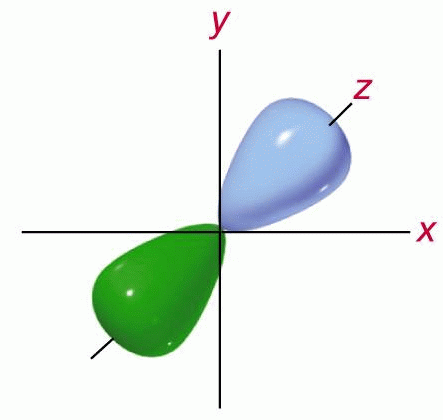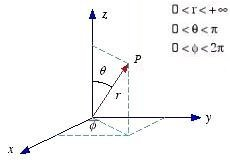In ROOT, option “sph” or “cyl” means the following:

you have a “coordinate sphere” or “coordinate cylinder” with radius ==
gStyle->GetLegoInnerR() and you simply map your surface (lego plot) from cartesian to sphere/cylinder. For example:[/img]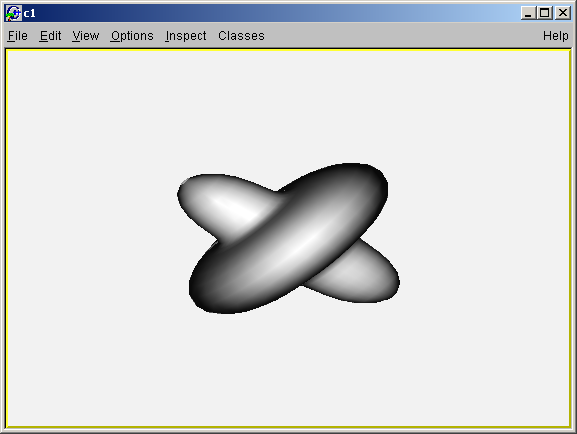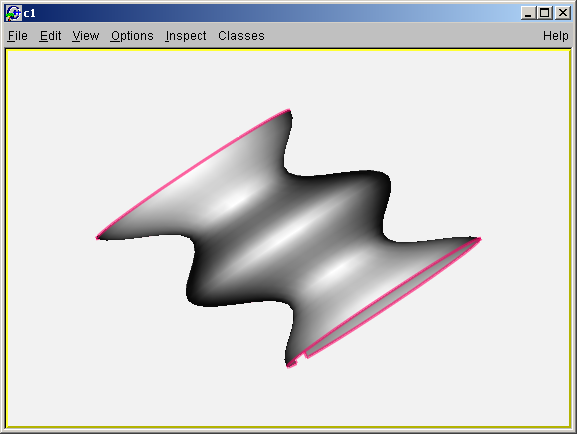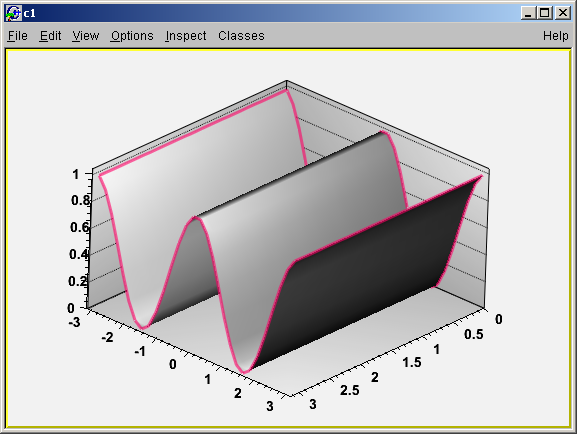How can I get the distribution that I want? Can you give me a quick example?

Thank you
Lutz

How can I get the distribution that I want? Can you give me a quick example?

Thank you
Lutz[/quote]

Do you want something like this :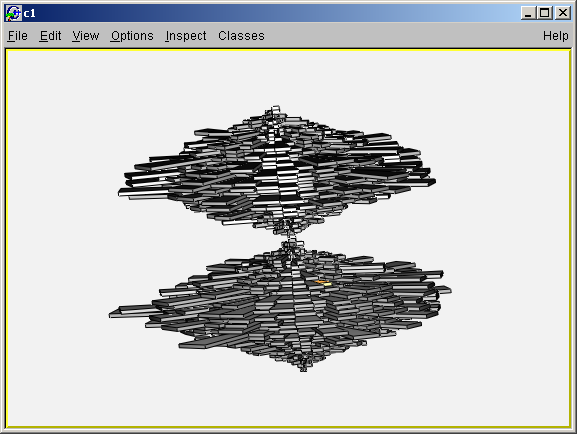Hello,

Thanks for your reply. This is exactly what I am looking for.
Could you sent me also the code, that created this distribution?

Thank you
Lutz

I think it is simply using option CYL instead of SPH. Isn’t it Timur ?

Yes, this is option cyl and different function.

``````void test2d()
{
//gStyle->SetCanvasPreferGL(true);
TH2D * twod = new TH2D("hpxpy","py vs px",36,0,3.14,72, -3.14, 3.14);
TF2 * f= new TF2("f","abs(sin(y))",0,3.14,-3.14,3.14);
twod->FillRandom("f", 2000000);
gStyle->SetLegoInnerR(0.01);
twod->SetFillColor(kGreen);
//twod->Draw("gllegocyl");
twod->Draw("legocyl");
} ``````